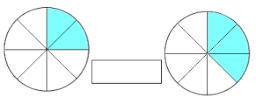# Equations: 43501

Solve two equations:

7 * (x + 1) - (3x + 1) = 21/2

a / 3-a + 6/12 = 2a-4

x =  1.125
a =  1.6875

### Step-by-step explanation:

7·(x+1)-(3·x+1)=21/2

8x = 9

x = 9/8 = 1.125

x = 9/8 = 1.125

Our simple equation calculator calculates it.

a/3-a+6/12=2·a-4

32a = 54

a = 54/32 = 1.6875

a = 27/16 = 1.6875

Our simple equation calculator calculates it.Did you find an error or inaccuracy? Feel free to write us. Thank you!

Tips for related online calculators
Need help calculating sum, simplifying, or multiplying fractions? Try our fraction calculator.
Do you have a linear equation or system of equations and looking for its solution? Or do you have a quadratic equation?